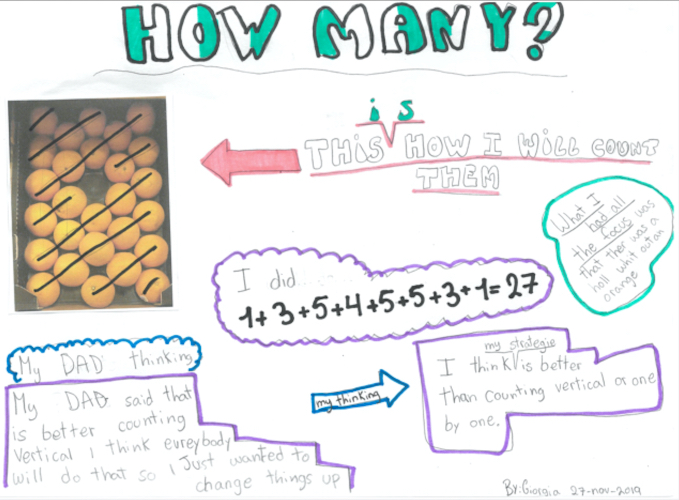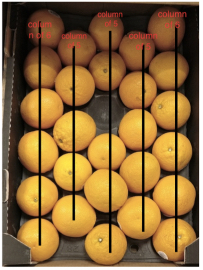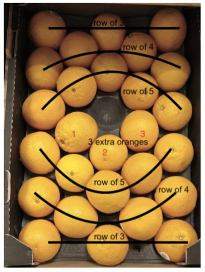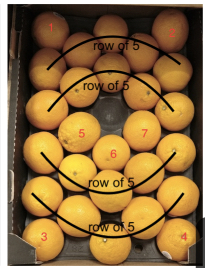#### You may also like### Month Mania

Can you design a new shape for the twenty-eight squares and arrange the numbers in a logical way? What patterns do you notice?### Noah

Noah saw 12 legs walk by into the Ark. How many creatures did he see?### Writing Digits

Lee was writing all the counting numbers from 1 to 20. She stopped for a rest after writing seventeen digits. What was the last number she wrote?

# How Many?

##### Age 5 to 11 Challenge Level:

We had a good number of solutions sent in to this task. These first ones are from Meavy C of E Primary School.

Samuel and Lucy
The first one. I spotted that it is an array and then I worked it out by counting the top line and the side line. Then I multiplied them. Like 4 x 5 = 20.
The last one we know we have 6 tubs and they mainly have around 50 to 60. Then we multiplied to get around 350 to 360.

Amir and Grace
Peaches: We saw there were 5 rows of 4 and 4 x 5 is 20.
Raspberries: there isn't really an answer. Say there's 20 in a pot 6 x 20 is 120.

Edith and Joe
Oranges: It would be about 27 because 3 x 5=15 then add 6 which equals 21 then adding on to that add another 6 which equals 27.
Peaches: There are 20 peaches here because 5 x 4 = 20.

Aoife
It equals 20 because 5 x 4 = 20.I counted the rowes and on the side row there 5. On the bottom 4.

Samuel and Lucy
I made it symmetrical and counted each side and it turned out to be 5 + 6 + 5 + 6 = 22. I added the middle line so it was 22 + 6 = 28. Then I subtracted one from the current answer 28. So the answer was 27 (when you do 28 - 1 = 27).

Then we had these three solutions from Kuala Lumpur Alice Smith School in Malaysia:

Coco and Seraphina
For the first one, you count all of the peaches, and get 20, then for the oranges, you count all of them as well, and get 37. Finally, you estimate, and get thirty approx, and times it by six, since there are six boxes. In total, you get 180 raspberries. We estimated 30, since there are about ten in each layer, and there are three layers.

Mia
For the raspberries my estimate for 1 box is: 39. My method was to take a screen shot then count the raspberries with dots. I knew how to separate the raspberries by looking at the lines. Then I did 39 x 6 = 234.

Hannah
So for the first one I just counted vertically and horizontally and multiplied them to get 20.
For the second I counted from top to bottom and got 27.
For the last one I took screenshots and crossed off the raspberries. I found a reasonable half way point of the numbers I'm guessing it might be 35.
PS: I didn’t go further than that.

Kayan from St. Martins School sent in the following:

1) 5 rows, each with 4 peaches so 5 x 4 = 20 altogether
2) We saw a pattern. Ignoring the hole, 3 columns of 6 oranges and 2 columns of 5 oranges.
So 6 x 3 = 18. 5 x 2 = 10.
18 + 10 = 28
Then we need to take 1 away for the hole. So 27 oranges.
3) We assume each box has the same number of raspberries and we count the berries in one box as 36.
So we need to do 6 x 36. We first do 6 x 30 = 180 and then we add on 6 x 6 = 36. 180 + 36 = 216

We had the following sent in from The International School of Brussels:Simran from Maurice Hawk School USA sent in this last piece of work together with three pictures:

The peaches are arranged as an array of 5 x 4, so there are 20 peaches. We can also count them as 5 rows of 4 each or 4 columns of 5 each, which makes 20 peaches either way we count.

The oranges were an interesting case. I stared at them for a while, there are a few different ways to count them. In the first way, there are two outer columns of 6 each and three inner columns of 5 each, so 27 oranges (6 x 2 + 5 x 3 = 27).
In the second way, there are rows of 3, 4 and 5 oranges and the pattern repeats on the lower side of the crate in reverse order and there are 3 extra oranges.
In the third way, there are four rows of 5 oranges each, 4 oranges in each corner and 3 extra oranges in the middle of the arrangement.
Any way you count there are 27 oranges but I think the first way is best as it does not leave any extra oranges to count later (see the pictures below).

The raspberry containers looked like quarter pound boxes. When I searched how many berries could be there, the answer was anywhere between 30 to 40 berries. So my estimate is that all the boxes of raspberries displayed contain between 180 to 240 berries.Thank you all for all the submissions that came in to us. It was good to read about the variety of ways you worked.